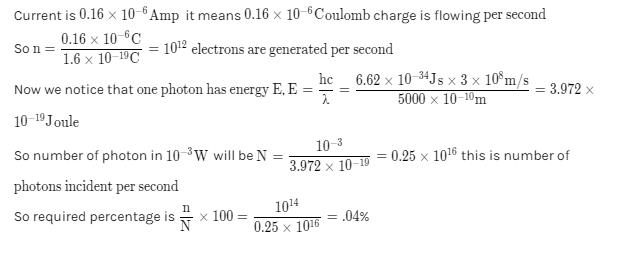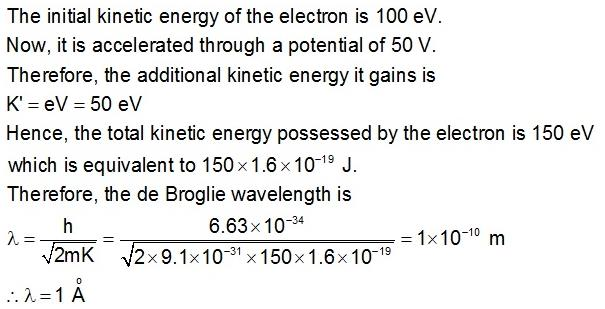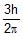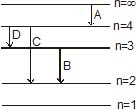Courses

# Test: Modern Physics (Competition Level 1)

## 30 Questions MCQ Test Physics Class 12 | Test: Modern Physics (Competition Level 1)

Description
This mock test of Test: Modern Physics (Competition Level 1) for Class 12 helps you for every Class 12 entrance exam. This contains 30 Multiple Choice Questions for Class 12 Test: Modern Physics (Competition Level 1) (mcq) to study with solutions a complete question bank. The solved questions answers in this Test: Modern Physics (Competition Level 1) quiz give you a good mix of easy questions and tough questions. Class 12 students definitely take this Test: Modern Physics (Competition Level 1) exercise for a better result in the exam. You can find other Test: Modern Physics (Competition Level 1) extra questions, long questions & short questions for Class 12 on EduRev as well by searching above.
QUESTION: 1

Solution:
QUESTION: 2

### 10-3 W of 5000 Å light is directed on a photoelectric cell. If the current in the cell is 0.16 mA, the percentage of incident photons which produce photoelectrons, is

Solution:QUESTION: 3

### If the frequency of light in a photoelectric experiment is doubled, the stopping potential will

Solution:
QUESTION: 4

The stopping potential for the photo electrons emitted from a metal surface of work function 1.7eV is 10.4 V. Identify the energy levels corresponding to the transitions in hydrogen atom which will result in emission of wavelength equal to that of incident radiation for the above photoelectric effect

Solution:
QUESTION: 5

When a photon of light collides with a metal surface, number of electrons, (if any) coming out is

Solution:
QUESTION: 6

A point source of light is used in photoelectric effect. If the source is removed farther from the emitting metal, the stopping potential

Solution:
QUESTION: 7

A point source causes photoelectric effect from a small metal plate. Which of the following curves may represent the saturation photocurrent as a function of the distance between the source and the metal?

Solution:

Saturation current is the maximum current possible and it will be directly proportional to the number of number of electrons falling on collector plate per second which depend on number of photons
incident on the cathode as one photon contribute in one electron and the number of photons is actually
proportional to intensity which varies
As intensity I∝1/r2​, where r is the distance
So the correct graph will be decreasing with power2 of distance and it will be rapidly decreasing with a higher value of r.

QUESTION: 8

The maximum kinetic energy of photoelectrons emitted from a surface when photons of energy 6 eV fall on it is 4eV. The stopping potential is Volts is

Solution:
QUESTION: 9

Radiation of two photon energies twice and five times the work function of metal are incident sucessively on the metal surface. The ratio of the maximum velocity of photoelectrons emitted is the two cases will be

Solution:
QUESTION: 10

Cut off potentials for a metal in photoelectric effect for light of wavelength l1, l2 and l3 is found to be V1, V2 and V3 volts if V1, V2 and V3 are in Arithmetic Progression and l1, l2 and l3 will be

Solution:
QUESTION: 11

Photons with energy 5eV are incident on a cathode C, on a photoelectric cell. The maximum energy of the emitted photoelectrons is 2eV. When photons of energy 6eV are incident on C, no photoelectrons will reach the anode A if the stopping potential of A relative to C is

Solution:
QUESTION: 12

By increasing the intensity of incident light keeping frequency (v > v0) fixed on the surface of metal

Solution:
QUESTION: 13

In a photoelectric experiment, electrons are ejected from metals X and Y by light of intensity I and frequency f. The potential difference V required to stop the electrons is measured for various frequencies. If Y has a greater work function than X ; which one of the following graphs best illustrates the expected results ?

Solution:
QUESTION: 14

A image of the sun of formed by a lens of focal-length of 30 cm on the metal surface of a photo-electric cell and a photo-electrci current is produced. The lens forming the image is then replaced by another of the same diameter but of focal length 15 cm. The photo-electric current in this case is

Solution:
QUESTION: 15

A proton and an electron are accelerated by same potential difference have de-Broglie wavelength lp and le.

Solution:
QUESTION: 16

An electron with initial kinetic energy of 100eV is acceleration through a potential difference of 50V. Now the de-Broglie wavelength of electron becomes.

Solution:QUESTION: 17

If h is Planck's constant is SI system, the momentum of a photon of wavelength 0.01 Å is

Solution:
QUESTION: 18

The angular momentum of an electron in the hydrogen atom is. Here h is Planck's constant. The kinetic energy of this electron is

Solution:
QUESTION: 19

Consider the following electronic energy level diagram of H-atom : Photons associated with shortest and longest wavelengths would be emitted from the atom by the transitions labelled.Solution:
QUESTION: 20

If the electron in a hydrogen atom were in the energy level with n = 3, how much energy in joule would be required to ionise the atom ? (Ionisation energy of H-atom is 2.18 × 10-18 J)

Solution:
QUESTION: 21

In a hydrogen atom, the electron is in nth excited state. It may come down to second excited state by emitting ten different wavelengths. What is the value of n

Solution:
QUESTION: 22

Difference between nth and (n + 1)th Bohr's radius of `H' atom is equal to it's (n _ 1)th Bohr's radius. The value of n is

Solution:
QUESTION: 23

The electron in a hydrogen atom makes transition from M shell to L. The ratio of magnitudes of initial to final centripetal acceleration of the electron is

Solution:
QUESTION: 24

The electron in a hydrogen atom makes a transition n1 → n2 whose n1 and n2 are the principal quantum numbers of the two states. Assume the Bohr model to be valid. The frequency of orbital motion of the electron in the initial state is 1/27 of that in the final state. The possible values of n1 and n2 are

Solution:
QUESTION: 25

The radius of Bohr's first orbit is a0. The electron in nth orbit has a radius

Solution:
QUESTION: 26

The ionisation potential of hydrogen atom is 13.6 volt. The energy required to remove an electron from the second orbit of hydrogen is :

Solution:
QUESTION: 27

In a sample of hydrogen like atoms all of which are in ground state, a photon beam containing photons of various energies is passed. In absorption spectrum, five dark lines are observed. The number of bright lines in the emission spectrum will be (Assume that all transitions take place)

Solution:
QUESTION: 28

When a hydrogen atom, initially at rest emits, a photon resulting in transition n = 5 → n = 1, its recoil speed is about

Solution:
QUESTION: 29

Consider the spectral line resulting from the transition n = 2 → n = 1 in the atoms and ions given below. The shortest wavelength is produced by :

Solution:
QUESTION: 30

In an atom, two electrons move around the nucleus in circular orbits of radii R and 4R. The ratio of the time taken by them to complete one revolution is : (neglect electric interaction)

Solution: